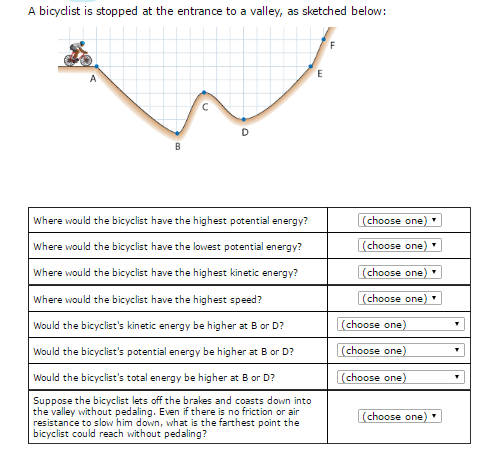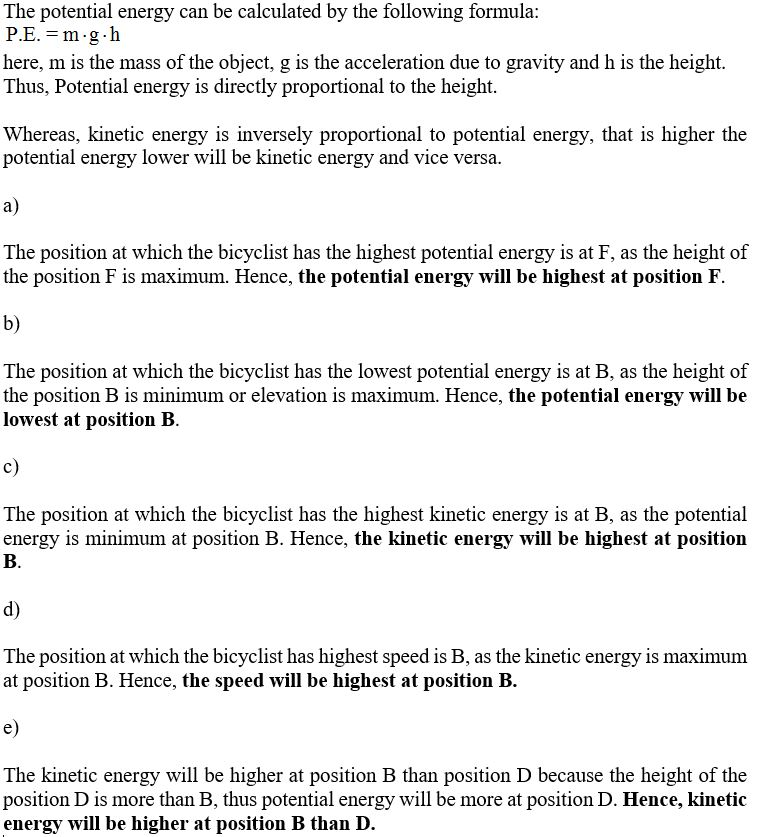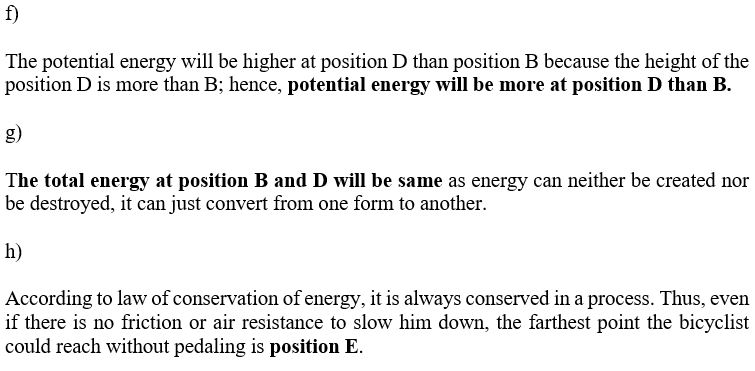# A bicyclist is stopped at the entrance to a valley. as sketched below:A bicyclist is stopped at the entrance to a valley, as sketched below: Where would the bicyclist have the highest potential energy? Where would the bicyclist have the lowest potential energy? Where would the bicyclist have the highest kinetic energy? Where would the bicyclist have the highest speed? Would the bicyclist’s kinetic energy be higher at B or D? Would the bicyclist’s potential energy be higher at B or D? Would the bicyclist’s total energy be higher at B or D? Suppose the bicyclist lets off the brakes and coasts down into the valley without pedaling. Even if there is no friction or air resistance to slow him down what is the farthest point the bicyclist could reach without pedaling?

The potential energy can be calculated by the following formula here, m is the mass of the object, g is the acceleration due to gravity and h is the height. Thus, Potential energy is directly proportional to the height Whereas, kinetic energy is inversely proportional to potential energy, that is higher the potential energy lower will be kinetic energy and vice versa a) The position at which the bicyclist has the highest potential energy is at F, as the height of the position F is maximum. Hence, the potential energy will be highest at position F. b) The position at which the bicyclist has the lowest potential energy is at B, as the height of the position B is minimum or elevation is maximum. Hence, the potential energy will be lowest at position B c) The position at which the bicyclist has the highest kinetic energy is at B, as the potential energy is minimum at position B. Hence, the kinetic energy will be highest at position d) The position at which the bicyclist has highest speed is B, as the kinetic energy is maximum at position B. Hence, the speed will be highest at position B e) The kinetic energy will be higher at position B than position D because the height of the position D is more than B, thus potential energy will be more at position D. Hence, kinetic energy will be higher at position B than D
f) The potential energy will be higher at position D than position B because the height of the position D is more than B; hence, potential energy will be more at position D than B. g) The total energy at position B and D will be same as energy can neither be created nor be destroyed, it can just convert from one form to another. h) According to law of conservation of energy, it is always conserved in a process. Thus, even if there is no friction or air resistance to slow him down, the farthest point the bicyclist could reach without pedaling is position EAlso Read :   if f (x)=x then f'(5) =?